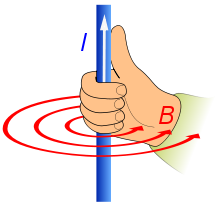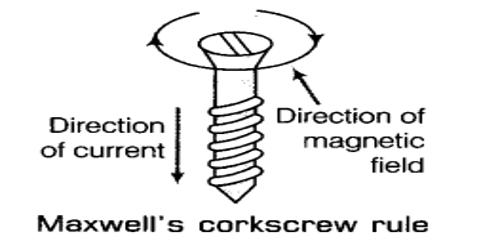Direction of current and magnetic lines of forceThe direction of electric current and magnetic lines of force can be determined by the help of following rules:

Right Hand Thumb Rule

Right Hand Thumb Rule can be used for straight current carrying conductor.According to this rule, if we imagine to hold a straight current carrying conductor such that the thumb points towards the direction of the current then the direction of the magnetic lines of force is given by the tips of the curled fingers encircling the conductor.

Maxwell's cork screw rule

This rule is also applicable for straight current carrying conductor.According to this rule, if we imagine the forward motion of the right handed cork screw to be the direction of the current then the direction of the rotation of the screw gives the direction of the magnetic lines of force around the conductor.

Right hand fist rule

This rule is also used for straight current carrying conductor and is similar to right hand thumb rule.According to this rule, if we curl the fingers in the direction of the current in circular loop then the direction of magnetic field lines is represented by the thumb.